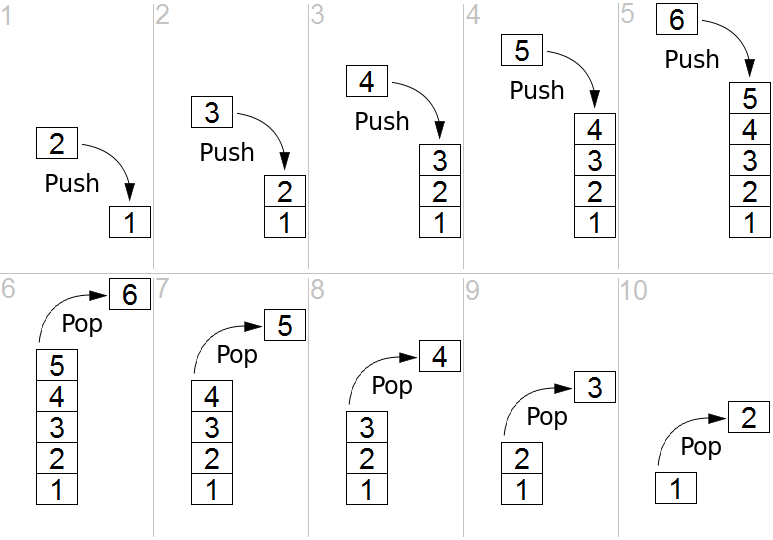# A Stack data structure in TypeScript

The JavaScript `Array` has everything to acts as a nice `Stack` structure, the only thing is missing is a `peek()` method. In this blog I'll discuss two ways of implementing a stack based on the array.

## What's a stack?

A picture says more than words:A stack is a LIFO structure: last in, first out. Imagine a stack of plates. Source: Stack (abstract data type)

Let's start with defining the what a stack should look like and let's keep it as close to what the `Array` already gives us:

``````interface Stack<T> {
push(item: T): number
pop(): T | undefined
peek(): T | undefined
[Symbol.iterator](): Iterator<T>
}
``````

Note: I've included the iterator to support spreading without having to cast.

## Module augmentation

The `push`, `pop`, `length` and `iterator` are already present on the `Array` object. The only thing we miss is the `peek` object. Let's use TypeScript module augmentation to add the `peek` feature to the array:

``````//!file: m-stack.ts

export interface Stack<T> {
push(item: T): number
pop(): T | undefined
peek(): T | undefined
[Symbol.iterator](): Iterator<T>
}

declare global {
interface Array<T> {
peek(): T
}
}

if (!Array.prototype.peek) {
Array.prototype.peek = function <T>(): T {
return this.length == 0
? undefined
: this[this.length - 1]
}
}
``````

You can use it like this:

``````import { Stack } from "./m-stack"
import "./m-stack"

let stack: Stack<string> = []
stack.push("kaas")
stack.push("is", "heel")
stack.push("lekker")

console.log(stack.length, stack.peek());
stack.pop()
console.log(stack.length, stack.peek());``````

When we forget `import "./m-stack"`, we'll get a runtime error: `TypeError: stack.peek is not a function`! Took me some time to figure out why.

Pro:

• I like the fact that we can declare an array and just cast it to `Stack<T>` and work with it.

Con:

• I really don't like the way we need to wire this up: it looks like we're importing the same thing twice.
• It extends the prototype, a technique some would call: prototype pollution. It looks like Node.js make this type of pollution more scoped.

## A class wrapper

If we don't want to pollute the array, we could create our own `Stack` class based on the `Array`:

``````//!file: n-stack.ts

export class Stack<T> {
private items: T[]

constructor(iterable: Iterable<T> = []) {
this.items = [...iterable]
}

pop = () => this.items.pop()
push = (...items: T[]) => this.items.push(...items)
peek = () =>
this.length == 0
? undefined
: this.items[this.length - 1]

get length() {
return this.items.length
}

*[Symbol.iterator]() {
for (let item of this.items) yield item
}
}
``````

Usage is similar:

``````import { Stack } from "./n-stack"

let stack = new Stack<string>()
stack.push("kaas")
stack.push("is", "heel")
stack.push("lekker")

console.log(stack.length, stack.peek());
stack.pop()
console.log(stack.length, stack.peek());

console.log([...stack])``````

Pro:

• It uses the same API as the `Array` object so it should feel familiar.
• It does not suffer from prototype pollution.

Con:

• Each stack is instantiated as a new object.
• We need to use the the iterator to get our objects back.

## Bonus: Queue

If you want a queue in the same way, just switch the `pop` for `shift`. The `peek` is always the first items of the array.

``````export class Queue<T> {
private items: T[]

constructor(iterable: Iterable<T> = []) {
this.items = [...iterable]
}

shift = () => this.items.shift()
push = (...items: T[]) => this.items.push(...items)
peek = () =>
this.length == 0
? undefined
: this.items

get length() {
return this.items.length
}

*[Symbol.iterator]() {
for (let item of this.items) yield item
}
}``````

## Conclusion

Implementing a Queue or a Stack in TypeScript is pretty straightforward. The heavy lifting has already been done by the native `Array` class. My preference goes to the class-implementation as it does not pollute and is easy to use. I would advise to stay as close to the `Array` API as possible.

expand_less